# Analysis and Simulation of Hydromagnetic Nano-Fluid Flow Passing an Inclined Heated Sheet

Analysis and Simulation of Hydromagnetic Nano-Fluid Flow Passing an Inclined Heated Sheet

Uchenna A. UkaKelvin O. Agbo Ekakitie Omamoke Emeka Amos Edwin D. Keneke

Department of Basic Sciences, School of Science and Technology, Babcock University, P.M.B 4003, Ilishan-Remo 121003, Ogun State, Nigeria

Department of Mathematics, Faculty of Science, Bayelsa Medical University, P.M.B. 178, Yenagoa, Nigeria

Department of Mathematics, Rivers State University, Port-Harcourt 500101, Rivers Sate, Nigeria

Department of Physics, Faculty of Science, Bayelsa Medical University, P.M.B. 178, Yenagoa, Nigeria

Corresponding Author Email:
ukau@babcock.edu.ng
Page:
1386-1396
|
DOI:
https://doi.org/10.18280/ijht.400605
11 September 2022
|
Accepted:
21 October 2022
|
Published:
31 December 2022
| Citation

OPEN ACCESS

Abstract:

The examination of nano-fluid in recent time has been encouraging just as its research interest cuts across some disciplines such as mathematics, mechanical, chemical, civil engineering, physics, earth and natural sciences. Its applicability in the industrial and technological advancement helps in controlling the rate at which heat is conducted and diffuses in a given medium, as well as improving thermal transportation through convection, radiation and conduction. In this work, the analysis of hydromagnetic nano-fluid flow past an inclined heated surface with temperature dependent non-uniform heat source/sink and thermal radiation under heat and mass transmission perspective is considered. Adequate similarity variables are employed in recovering the nonlinear coupled ordinary differential equations (ODEs) from the partial differential equations (PDEs) which describe the equation of the boundary layer. The transformed equations (ODEs) are addressed both analytically and numerically with the aid of regular series approximation technique and Wolfram Mathematica package. The numerically simulated obtained results are graphically presented with legends. The computations opined that velocity declines while energy increases as the magnetic field number enhances. Similarly, improvement on angle of inclination, breeds velocity deceleration. Furthermore, the rate of energy transfer increases as the temperature dependent non-uniform parameters due to thermal generation enhances. In addition, the nanoparticle concentration decreases with increasing values of Schmidt and thermal radiation factors.

Keywords:

free convection, hydrodynamic, nanoliquid, series approximation, similarity transformation, thermodiffusion, diffusivity, nanoparticle

1. Introduction

The study of fluid dynamics has attracted the research interest of several scientific researchers due to its wide applications which ranges from the computation of forces and moments on aircraft, rate of mass flow of petroleum passing through oil pipelines, forecasting of weather variabilities, forming fission armament explosion etc. Similarly, nano-fluid exhibits thermos-physical features like enhanced heat conduction and diffusion as well as heat transmission through convection and radiation. Meanwhile, nano-fluid is a blend of conventional fluid such as water, ethylene glycol (ECG), oil etc., and particles of metal, oxides and molten liquids. Ordinarily, the conventional fluids also referred to as base fluids are conductors of heat but in order to improve their thermos-physical conductivity, the introduction of nanoparticles becomes expedient. However, fluids exist in form of liquids, air, gasses and each of them enjoys a whole lot of applications in several areas such as in aerospace, automotive, processing of food and beverages, production of glasses and chemicals, electronics, calibration gas mixtures for controlling the environment due to coal fired power generating stations, leather processing, mining, medicals, high-voltage insulations, cutting and welding activities and a good component of refrigeration process etc. Similarly, the application of magnetic field in a nanoparticle filled system, for particles that are electrically conducting produces magnetic nanoparticles. The magnetic nanoparticles remarkably increase the thermal conductivity of the base fluid. However, the blending of different nano-sized particles of various materials in form of metallic and non-metallic oxides immersed in a convectional fluid such as H2O, as a result of their capacities as heat removers and coolants have been analysed by several scholars in the past, Godson et al. , Keblinski , Ozerinc et al. , Wen et al. . The magnetic nano-fluids which are made up of colloidal blends of highly paramagnetic nanoparticles and dispersed in a non-magnetic transporting fluid create a distinct type of nano-fluids with magnetic fluid characteristics . In such mixtures, properties such as fluid movement, random motion of nanoparticles as well as thermal transmitting processes can be tamed by the application of magnetic fields. Also with this form of fluids, the possibility of accumulation of the particles owing to Vander wall impact and magnetic interactions among the particles exist. Thus, to control this situation, the coating of the nanoparticles through the use of a surfactant layer is carried out. However, a compound that reduces the surface tension among dual liquids or a liquid-solid mix is termed surfactants. Bio-medically, magnetic nano-fluids compliments to the modern procedures for the treatment of cancer patients by using them in distributing the radiation or drugs to different affected body areas. This feat can be attributed to the adhesiveness property possessed by the magnetic nanoparticles. Hence, surf-acted magnetic nano-fluids having lauric acid are deployed during magnetic drug testing, biomolecules restriction etc. . Also, due to the existence of ammonia in water in different areas of the earth, the well-being of mammals are subjected to risk, especially when the mass of such a gas surpasses a particular value . Nonetheless, magnetic nano-fluids also have applicability in the areas of leakage of free turning seal . In vacuum installation systems, a highly powered electric controlling devices and liquefied gas pumps magnetic nano-fluids are very useful. Therefore, the usage of magnetic seals exists in computer hard disk systems, high vacuum schemes, chemical machineries, oil refineries .

However, applications of thermal and mass transmissions are vital in the processes leading to the production of synthetic rubber, plastic, Textile, industrial packaging of agricultural produce, hydroelectric power, metal fabrication and annealing as well as in mining processes etc. Thus, a work on the impact of chemical reactions MHD visco-stretched fluid flow past a perpendicular elastic plate due to thermal generation and absorption was done by Jena et al. . Through the application of the Runge-Kutta fourth order technique with the shooting method, they were able to solve the problem numerically. Thus, they established that chemical reaction, Prandtl and Soret parameters were accountable for the growth in the rate of heat passage at the plate. Similarly, Gurram et al.  investigated the MHD Casson fluid flow through an inclined plate in the presence of radiation due to the effect of chemical reaction by implementing the perturbation technicality to obtain the analytical result. From their findings, the heat wall thickness becomes enhanced as the perturbation factor intensifies. With hall current, Raju  considered the significance of a chemical reaction and buoyancy impact on an unsteady MHD natural and forced convections flow with an unbounded perpendicular permeable sheet. He embraced the Galerkein finite element scheme in solving the problem and infers that velocity distribution rises as hall and Grashof parameters of thermal and concentration transmissions also intensify. Venkateswarlu et al.  studied the role of mass transport on nonlinear MHD boundary layer flow of a viscid non-compressible fluid along a nonlinear leaky elastic plate in a permeable medium with heat source. Numerically, Bilal and Nazeer  investigated the non-Newtonian flow along a stratified elastic/lessening inclined plate due to an associated magnetic field and nonlinear convective flow. Their study was carried out by deploying the shooting approach and from their findings, it was captured that the local inertia coefficient is responsible for the declining nature of the velocity field. Nevertheless, the importance of MHD in the advancement of technology cannot be over-emphasised. Hence, the examination on the effect of MHD flow transitory along an exponentially inclined upright sheet of first-order chemical reaction due to changing mass diffusion and heat radiation was observed by Usharami et al. . The utilization of the Laplace transmute process enabled the solution of their problem. Thus, the obtained result is indicative of the fact that the expense of intensification of the affinity edge of an effected attraction region leads to the receding of the speed profile.

In this work, we shall formulate a mathematical flow model governing the fluid flow and apply the regular series approximation technique in solving the problem. Then, the examination of the effect of the temperature dependent non-uniform heat source/sink parameters due to thermal source and mass transmission on hydrodynamic natural convective stream over an inclined sheet with magnetic field are investigated after solving the transformed dimensionless models analytically together with numerical simulation. Furthermore, the impacts of different fluid physical parameters on the momentum, energy and concentration specie (nanoparticles) distributions are depicted with graphs and legends. Thus, the results are discussed in details with an informing conclusion drawn.

2. Mathematical Formulation of the Problem

This section analyses a steady hydromagnetic flow of a viscid, non-compressible fluid with electrical conductivity characteristics, over an inclined sheet in the presence of a critical angle γ towards the perpendicular direction. The assumption is that the x direction which is analogous to the inclined sheet is related to the path of the flow and the normal is represented by the y axis. An introduction of a uniform strength magnetic field coefficient Bo acts in the flow direction. The magnetic Reynolds factor gives an estimate of the relative effects of induction of magnetic field by the motion of a conducting medium. The fluid we considered is slightly conducting, so the ratio of inductance to diffusion is less than unity (i.e., the magnetic Reynolds number Re<<1), hence the induced magnetic field is negligible in comparison with the applied magnetic field. Also, all the properties of the fluid remain constant apart from the impact of the density disparity in line with energy as well as nanoparticle specie concentration in the quantity for the body force. At a non-varying temperature $T_w$, the wall is maintained more than at temperature of the conducting fluid far from the wall $T_{\infty}$. However, $C_w$ and $C_{\infty}$ are the nanoparticle concentration at the wall and far away from the wall. According to Hakeem et al. , the temperature dependent non-uniform heat source/sink is given as:

$q^{\prime \prime \prime}=\frac{x R a_x^{\frac{1}{2}}}{2 x^2}\left[G\left(T_s-T_{\infty}\right) z^{\prime}(\eta)+H\left(T-T_{\infty}\right)\right]$                 (1)

## 1.png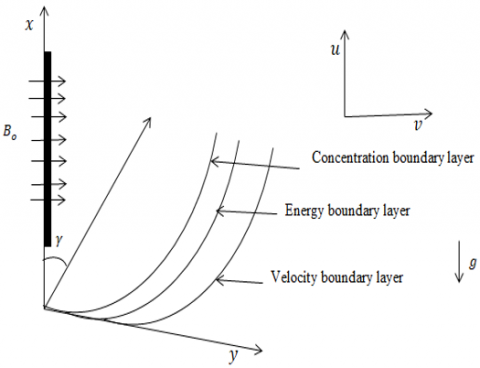Figure 1. Physical flow geometry with coordinates

Thus, the flow geometry is given in Figure 1 while the mathematical models governing the fluid flow analogy include:

$\frac{\partial u}{\partial x}+\frac{\partial v}{\partial y}=0$            (2)

$\left.\begin{array}{c}\rho_f\left(u \frac{\partial u}{\partial x}+v \frac{\partial v}{\partial y}\right)=v \frac{\partial^2 u}{\partial y^2}-\frac{\sigma B_o^2}{\rho} u+ \\ \left(1-C_{\infty}\right) \rho_{\infty} g \beta_T\left(T-T_{\infty}\right) \cos \gamma+ \\ \left(\rho_p-\rho f_{\infty}\right) g \beta_c\left(C-C_{\infty}\right) \cos \gamma\end{array}\right\}$              (3)

$u \frac{\partial T}{\partial x}+v \frac{\partial T}{\partial y}=\alpha \frac{\partial^2 T}{\partial y^2}+\tau D_B\left(C-C_{\infty}\right)\left(T-T_{\infty}\right)+\frac{\sigma B_o^2}{\rho}\left(T-T_{\infty}\right)-\frac{1}{(\rho C)_f} \frac{\partial q r}{\partial y}+\frac{1}{(\rho C)_f} q^{\prime \prime \prime}$                  (4)

$u \frac{\partial C}{\partial x}+v \frac{\partial C}{\partial y}=D_s \frac{\partial^2 C}{\partial y^2}+\frac{D_T}{T_{\infty}}\left(T-T_{\infty}\right)$            (5)

Dependent on the following boundary conditions

$\left.\begin{array}{c}u=U_w, v=-s_o, T=T_s, C=C_s \text { at } y=0 \\ u=v=0, T \rightarrow T_{\infty}, C \rightarrow C_{\infty} \text { as } y \rightarrow \infty\end{array}\right\}$                (6)

However, the negative sign existing in $v=-s_o$ infers that the existence of suction is in the same direction with the sheet.

3. Method of Solution

The processes adopted in the solution of the problem under consideration are as follows:

• The mathematical models describing fluid movement which appears in partial differentials are transformed into joined ordinary differential equations.
• The boundary conditions to which the fluid problem is also converted to a dimensionless form.
• Mathematical definitions involving suction term, new independent variable, dimensionless velocity, energy and specie concentration are adopted.
• Due to convergent series solutions, solutions to the problem are presumed, differentiated and applied to the converted equations.
• Also, the mathematical definitions are differentiated up to third-order, substituted into the transformed mathematical models and simplified such that, new models are obtained. Also, the boundary conditions are changed again with the aid of the new definitions already mentioned above.
• Coefficients whose independent variables have equal index are equated while others with orders above two (2) are neglected.
• All the obtained equations are solved one after the other with their corresponding conditions at the boundary.
• The Mathematica and Wolfram codes are deployed in gaining the numerical solutions from analytical form.

According to Poddar et al. , the analogy of the Rosseland approximation in relation to the radiation heat fluctuation simplifies to

$\frac{\partial q r}{\partial y}=-\frac{4 \sigma_0 \partial T^4}{3 k_o \partial y}$          (7)

Such that $\sigma_0$ represents the Stefan-Boltzmann quantity. Thus, expanding the highest term in Eq. (7) with Taylor series without considering higher degrees results to

$T^4 \approx 4 T T_{\infty}^3-3 T_{\infty}^4$           (8)

Therefore, simplifying further and substituting into Eq. (4) above reduces to

$u \frac{\partial T}{\partial x}+v \frac{\partial T}{\partial y}=\alpha \frac{\partial^2 T}{\partial y^2}+\tau D_B\left(C-C_{\infty}\right)\left(T-T_{\infty}\right)+\frac{\sigma B_o^2}{\rho}\left(T-T_{\infty}\right)++\frac{1}{(\rho C)_f} q^{\prime \prime \prime}+\frac{16 \sigma_0 T_{\infty}^4 \partial^2 T}{3(\rho C)_f k_o \partial y^2}$                  (9)

The similarity variables needed for the transformation of Eqns. (2)-(4) and (9) are:

$\left.\begin{array}{rl}\varphi & =f(\eta) \sqrt{v x U_{\infty}}, \eta=\frac{y}{\sqrt{\frac{v x}{U_{\infty}}}} \\ \theta(\eta) & =\frac{T-T_{\infty}}{T_s-T_{\infty}}, \emptyset(\eta)=\frac{C-C_{\infty}}{C_s-C_{\infty}}\end{array}\right\}$              (10)

Eqns. (3), (5), (6) and (9) are converted into coupled nonlinear ordinary differential models through the standard similarity conversion procedure, Uka et al.  by using Eq. (10) and the results are:

$\frac{d^3 f}{d \eta^3}+\frac{1}{2} f(\eta) \frac{d^2 f}{d \eta^2}-M \frac{d f}{d \eta}+G_h \theta(\eta) \cos \gamma+G_c \emptyset(\eta) \cos \gamma=0$         (11)

$\frac{d^2 \theta}{d \eta^2}(1+S)+\operatorname{Prh}(\eta) \frac{d \theta}{d \eta}+N b \theta(\eta) \emptyset(\eta)++M \theta(\eta)+G \frac{d f}{d \eta}+H \theta(\eta)=0$            (12)

$\frac{d^2 \emptyset}{d \eta^2}+\frac{1}{2} S c f(\eta) \frac{d \emptyset}{d \eta}+\frac{N t}{N b} \theta(\eta)=0$         (13)

$f(0)=k_0, f^{\prime}(0)=1, \theta(0)=1 \emptyset(0)=1$ at $\eta=0$

$f^{\prime}(\infty) \rightarrow 0, \theta(\infty) \rightarrow 0, \emptyset(\infty) \rightarrow 0$ as $\eta \rightarrow \infty$            (14)

with the following dimensionless measures,

$M=\frac{\sigma B_0^2 x}{\rho U_{\infty}}, G_h=\frac{\left(1-C_{\infty}\right) \rho_{\infty} g \beta_T\left(T_s-T_{\infty}\right)}{U_{\infty}^2}$,

$G_c=\frac{\left(\rho_p-\rho f_{\infty}\right) g \beta_c\left(C-C_{\infty}\right) x}{U_{\infty}^2}, N b=\frac{\tau D_s\left(C_s-C_{\infty}\right) U_{\infty}}{v x}$,

$P r=\frac{v}{\alpha}, G=H=\frac{C R a_x^{\frac{1}{2}} \mu}{2 x k U_{\infty}}, S=\frac{16 T_{\infty}^3 \sigma_0^*}{3 k^*}$,

$N t=\frac{\tau D_T\left(C_s-C_{\infty}\right) v x}{T_{\infty}}, S c=\frac{v}{D_s}, \gamma$ and $k_0=\frac{2 w}{\sqrt{\frac{v U_{\infty}}{x}}}$,

Denoting magnetic field number, convective heat Grashof parameter, convective mass Grashof parameter, random (Brownian) motion number, Prandtl parameter, temperature dependent non-uniform heat source/sink numbers, radiation parameter, thermophoresis number, Schmidt factor, angle of inclination and velocity suction parameters.

Due to the adopted approach of solution, we defined the following terms which involves a very small parameter, an independent variable, $\eta$ and non-dimensional momentum, energy and nanoparticle concentration as follows:

$\left.\begin{array}{c}\eta=\xi k_0, h(\eta)=k_0 h(\eta), \theta(\eta)=g(\eta), \\ \emptyset(\eta)=w(\eta), \delta=\frac{1}{k_0^2}\end{array}\right\}$              (15)

Thus Eq. (15) and its derivatives are substituted into Eqns. (11)-(14) and simplified to obtain the following:

$\frac{d^3 h}{d \eta^3}+\frac{1}{2} h(\eta) \frac{d^2 h}{d \eta^2}-M \frac{d h}{d \eta} \delta+G_h g(\eta) \cos \gamma \delta^2+G_c w(\eta) \cos \gamma \delta^2=0$              (16)

$\frac{d^2 g}{d \eta^2}(1+S)+\operatorname{Prh}(\eta) \frac{d g}{d \eta}+\operatorname{Nbg}(\eta) w(\eta) \delta+M g(\eta) \delta+G \frac{d h}{d \eta}+H g(\eta) \delta=0$            (17)

$\frac{d^2 w}{d \eta^2}+\frac{1}{2} S c \frac{d w}{d \eta} h(\eta)+\frac{N t}{N b} g(\eta) \delta=0$          (18)

$\left.\begin{array}{c}h(0)=1, h^{\prime}(0)=\delta, \theta(0)=1, \emptyset(0)=1 \\ h^{\prime}(\infty)=0, \theta(\infty)=0, \emptyset(\infty)=0\end{array}\right\}$         (19)

For the existence of solutions for Eqns. (16)-(18), the expansion in degrees of $\delta$ is applied. Therefore, the regular perturbation series technique is applied thus:

Let the solutions of Eqns. (16)-(18) be defined as:

$H(\eta)=1+\delta h_1(\eta)+h_2(\eta) \delta^2+O\left(\delta^3\right)+\cdots$         (20)

$\theta(\eta)=g_0+\delta g_1+O\left(\delta^2\right)+\cdots$         (21)

$\emptyset(\eta)=w_0+\delta w_1+O\left(\delta^2\right)+\cdots$     (22)

Putting Eqns. (20)-(22) and their differentials into Eqns. (16)-(19), and simplifying, the order of the infinitesimal parameters are examined and the coefficients of corresponding order (index) are equated in such a way that the non-dimensional models representing momentum, energy and nanoparticle concentrations in the index of zero, unity and quadratic are given below:

$\left.\begin{array}{c}\frac{d^2 g_0}{d \eta^2}(1+S)+\operatorname{Pr} \frac{d g_0}{d \eta}=0 \\ g_0(0)=1, g_0(\infty)=0\end{array}\right\}$              (23)

$\frac{d^2 w_0}{d \eta^2}+\frac{1}{2} S c \frac{d w_0}{d \eta}=0 ; w_0(0)=1, w_0(\infty)=0$                (24)

For the unity index, we have

$\left.\begin{array}{c}\frac{d^3 h_1}{d \eta^3}+\frac{1}{2} \frac{d^2 h_1}{d \eta^2}=0 h_1(0)=0, \\ h_1{ }^{\prime}(0)=1, h_1^{\prime}(\infty)=0\end{array}\right\}$              (25)

$\left.\begin{array}{c}\frac{d^2 g_1}{d \eta^2}(1+S)+\operatorname{Pr} \frac{d g_1}{d \eta}+\operatorname{Prh}_1(\eta) \frac{d g_0}{d \eta}+ \\ N b g_0(\eta) w_0(\eta)+M g_0(\eta)+G \frac{d h_1}{d \eta}+ \\ H g_0(\eta)=0 ; g_1(0)=0, g_1(\infty)=0\end{array}\right\}$                   (26)

$\left.\begin{array}{l}\frac{d^2 w_1}{d \eta^2}+\frac{1}{2} S c \frac{d w_1}{d \eta}+\frac{1}{2} S c \frac{d w_0}{d \eta} h_1(\eta)+ \\ \frac{N t}{N b} g_0(\eta)=0 ; w_1(0)=0, w_1(\infty)=0\end{array}\right\}$                    (27)

While in terms of the quadratic index, we obtained:

$\left.\begin{array}{c}\frac{d^3 h_2}{d \eta^3}+\frac{1}{2} \frac{d^2 h_2}{d \eta^2}+\frac{1}{2} h_1(\eta) \frac{d^2 h_1}{d \eta^2}-M \frac{d h_1}{d \eta}+ \\ G_h g_0(\eta) \cos \gamma+G_c w_0(\eta) \cos \gamma=0; \\ h_2(0)=0, h_2^{\prime}(0)=0, h_2^{\prime}(\infty)=0\end{array}\right\}$                    (28)

Therefore, solving Eqns. (23)-(28) by the application of the integrating factor method and substituting the solutions obtained into Eqns. (17), (18) and (19) respectively, yields:

\begin{aligned} H(\eta)=1 & +\delta\left(2 P_2+P_3 e^{-\frac{1}{2} \eta}\right)+\delta^2\left(-P_2 P_3 e^{-\frac{1}{2} \eta}\right. \\ & +\frac{1}{2}\left(P_3\right)^2 e^{-\eta}-2 M P_3 \eta e^{-\frac{1}{2} \eta} \\ & +\frac{G_h J_2 \cos \gamma}{(A)^2\left(A-\frac{1}{2}\right)} e^{-A \eta} \\ & +\frac{4 G_c H_2 \cos \gamma}{(S c)^2\left(\frac{1}{2} S c-\frac{1}{2}\right)} e^{-\frac{1}{2} S c \eta} \\ + & \left.2 P_4 \eta-P_4+2 P_5+P_6 e^{-\frac{1}{2} \eta}\right)\end{aligned}                      (29)

$H^{\prime}(\eta)=\delta\left(-\frac{1}{2} P_3 e^{-\frac{1}{2} \eta}\right)+\delta^2\left(\frac{1}{2} P_2 P_3 e^{-\frac{1}{2} \eta}-\right.$

$-\frac{1}{2}\left(P_3\right)^2 e^{-\eta}-2 M P_3 e^{-\frac{1}{2} \eta}+M P_3 \eta e^{-\frac{1}{2} \eta}-$

$\frac{G_h J_2 \cos \gamma}{A\left(A-\frac{1}{2}\right)} e^{-A \eta}-\frac{2 G_c H_2 \cos \gamma}{S c\left(\frac{1}{2} S c-\frac{1}{2}\right)} e^{-\frac{1}{2} S c \eta}+2 P_4-\left.\frac{1}{2} P_6 e^{-\frac{1}{2} \eta}\right)$                    (29a)

But the velocity is defined as:

$h^{\prime}(\eta)=H^{\prime}(\eta) k_0^2$

Hence, the velocity of the fluid transmission is stated thus:

\begin{aligned} h^{\prime}(\eta)=-\frac{1}{2} P_3 e^{-\frac{1}{2} \eta} & +\delta\left(\frac{1}{2} P_2 P_3 e^{-\frac{1}{2} \eta}-\frac{1}{2}\left(P_3\right)^2 e^{-\eta}\right. \\ & -2 M P_3 e^{-\frac{1}{2} \eta}+M P_3 \eta e^{-\frac{1}{2} \eta} \\ & -\frac{G_h J_2 \cos \gamma}{A\left(A-\frac{1}{2}\right)} e^{-A \eta} \\ & -\frac{2 G_c H_2 \cos \gamma}{S c\left(\frac{1}{2} S c-\frac{1}{2}\right)} e^{-\frac{1}{2} S c \eta}+2 P_4 \\ & \left.-\frac{1}{2} P_6 e^{-\frac{1}{2} \eta}\right)\end{aligned}                  (30)

Such that the following constants were obtained from the solution.

$A=\frac{P r}{1+s}, H_2=1, J_2=1, P_2=1, P_3=-2$,

$P_6=P_2 P_3-\left(P_3\right)^2-4 M P_3-\frac{2 G_h J_2 \cos \gamma}{A\left(A-\frac{1}{2}\right)}-\frac{4 G_c H_2 \cos \gamma}{S c\left(\frac{1}{2} S c-\frac{1}{2}\right)}$                (31)

The solution for the fluid energy infers:

$\left.\begin{array}{c}g(\eta)=J_2 e^{-A \eta}+\delta\left(-\frac{2 J_2 P_2}{(1+S)} \eta e^{-A \eta}\right. \\ +\frac{2 A J_2 P_3}{\left(A+\frac{1}{2}\right)(1+S)} e^{-\left(\frac{1}{2}+1\right) \eta} \\ -\frac{2 N b H_2 J_2}{S c\left(A+\frac{1}{2} S c\right)(1+S)} e^{-\left(\frac{1}{2} S c+A\right) \eta} \\ +\frac{M J_2}{A(1+S)} \eta e^{-A \eta}+\frac{G P_3}{\left(\frac{1}{2}-A\right)(1+S)} e^{-\frac{1}{2} \eta} \\ \left.+\frac{H J_2}{A(1+S)} \eta e^{-A \eta}+\frac{J_3}{A}+J_4 e^{-A \eta}\right)\end{array}\right\}$                    (32)

where,

$A=\frac{P r}{1+S}, H_1=0, H_2=1, P_1=0, P_2=1, P_3=-2, J_1=0$,

$J_2=1, J_3=0$,

$J_4=\frac{2 N b H_2 J_2}{S c\left(A+\frac{1}{2} S c\right)(1+S)}-\frac{2 A J_2 P_3}{\left(A+\frac{1}{2}\right)(1+S)}-\frac{G P_3}{\left(\frac{1}{2}-A\right)(1+S)}$,

Are constants obtained from the solution.

For the nanoparticle (specie) concentration, we have:

$\left.\begin{array}{c}w(\eta)=H_2 e^{-\frac{1}{2} S c \eta}+\delta\left[-H_2 P_2 \eta e^{-\frac{1}{2} S c \eta}\right. \\ +\frac{H_2 P_3 S c}{1+S c} e^{-\left(\frac{1}{2}+\frac{1}{2} S c\right) \eta}-\frac{N t J_2}{N b\left(A-\frac{1}{2} S c\right)} e^{-A \eta} \\ \left.+\frac{2 H_3}{S c}+H_4 e^{-\frac{1}{2} S c \eta}\right]\end{array}\right\}$                 (33)

with,

$A=\frac{P r}{1+S}, P_1=0, P_2=1, P_3=-2, H_1=0, H_2=1, H_3=0$,

$H_4=\frac{J_2=1}{N b\left(A-\frac{1}{2} S c\right)}-\frac{H_2 P_3 S c}{1+S c}$

The physical quantities of interest such as the skin friction, Nusselt and Sherwood quantities are as given below:

$\left.\begin{array}{c}h^{\prime \prime}(\eta)=k_0^3 H^{\prime \prime} \\ g^{\prime}(\eta)=g_0^{\prime}(\eta)+\delta g_1^{\prime}(\eta) \\ \Rightarrow N u=-\left(\frac{\partial g}{\partial \eta}\right)_{\eta=0} \\ w^{\prime}(\eta)=w_0^{\prime}(\eta)+\delta w_1^{\prime}(\eta) \\ \Rightarrow S h=-\left(\frac{\partial w}{\partial \eta}\right)_{\eta=0}\end{array}\right\}$                (34)

$h^{\prime \prime}(0)=\frac{1}{4} P_3 k_0+\frac{1}{k_0}\left(-\frac{1}{4} P_2 P_3+\frac{1}{2}\left(P_3\right)^2+2 M P_3+\frac{G_h J_2 \cos \gamma}{\left(A-\frac{1}{2}\right)}+\frac{G_c H_2 \cos \gamma}{\left(\frac{1}{2} S c-\frac{1}{2}\right)}+\frac{1}{4} P_6\right)$                    (35)

$-N u=A J_2-\frac{1}{\left(k_o\right)^2}\left[-\frac{2 J_2 P_2}{(1+S)}-\left(\frac{3}{2}\right) \frac{2 A J_2 P_3}{\left(A+\frac{1}{2}\right)(1+S)}+\frac{2 N b H_2 J_2}{S c(1+S)}+\frac{M J_2}{A(1+S)}-\frac{1}{2} \frac{G P_3}{\left(\frac{1}{2}-A\right)(1+S)}+\frac{H J_2}{A(1+S)}+A\right]$                    (36)

$S h=-\frac{1}{2} H_2 S c+\frac{1}{\left(k_o\right)^2}\left[-H_2 P_2--\left(\frac{1}{2}+\frac{1}{2} S c\right) \frac{H_2 P_3 S c}{1+S c}+\frac{A N t J_2}{N b\left(A-\frac{1}{2} S c\right)}-\frac{1}{2} H_3 S c\right]$             (37)

where, the primes i.e., $\left({ }^{\prime}\right)$ implies differential with respect to $\eta$.

4. Results and Discussion

4.1 Figures and tables

## 2.png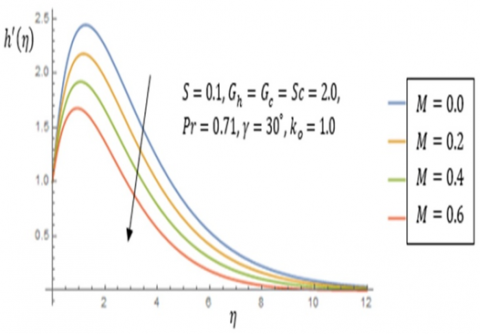Figure 2. Impact of magnetic field parameter, M on velocity

## 3.png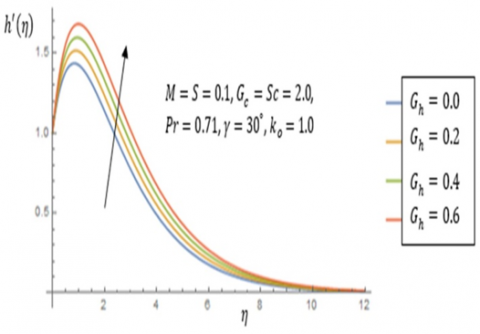Figure 3. Impact of modified convective heat Grashof strength, $G_h$ on velocity

## 4.png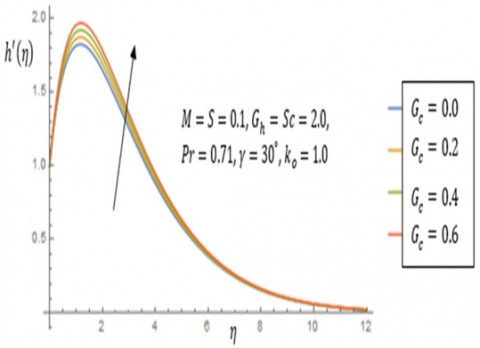Figure 4. Impact of modified convective specie Grashof strength, $G_c$ on velocity

## 5.png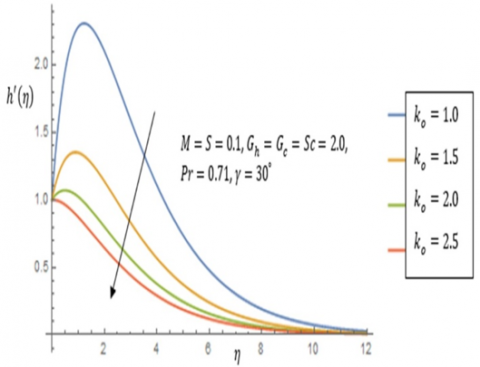Figure 5. Impact of suction parameter, $k_o$ on velocity

## 6.pngFigure 6. Impact of radiation, S on velocity

## 7.pngFigure 7. Impact of inclination angle, γ on velocity

## 8.png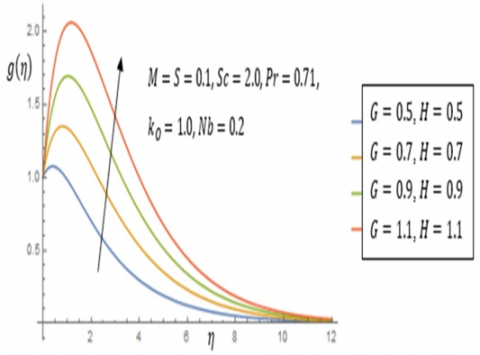Figure 8. Impact of heat dependent non-uniform numbers, G and H on temperature

## 9.png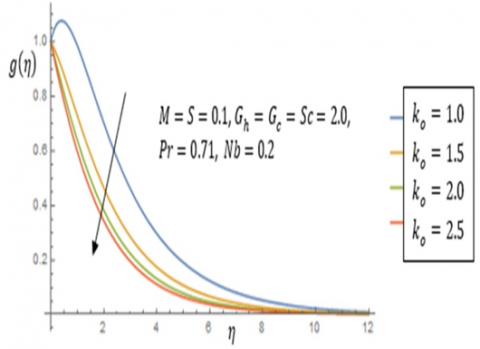Figure 9. Impact of velocity suction, $k_o$ on temperature

## 10.png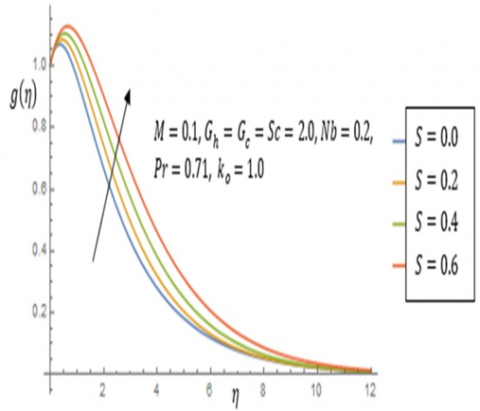Figure 10. Impact of radiation number, S on temperature

## 11.pngFigure 11. Impact of magnetic field factor, M on temperature

## 12.pngFigure 12. Impact of nanoparticle motion parameter, Nb on temperature

## 13.png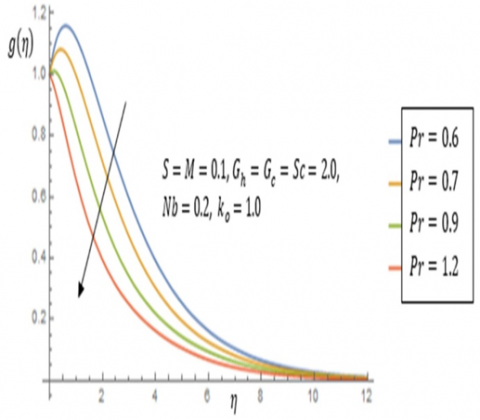Figure 13. Impact of Prandtl factor, Pr on temperature

## 14.pngFigure 14. Impact of velocity suction factor, $k_o$ on nanoparticle (specie) concentration

## 15.png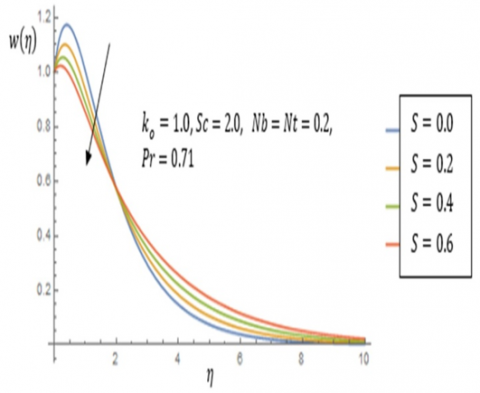Figure 15. Impact of radiation number, S on nanoparticle (specie) concentration

## 16.png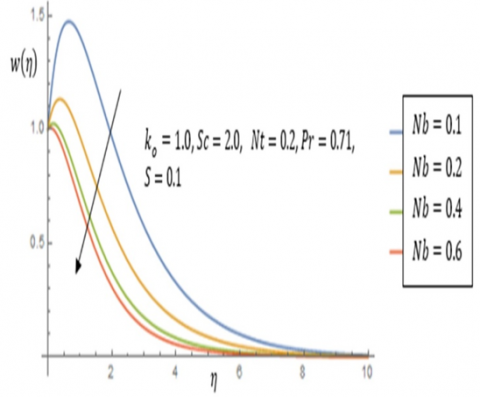Figure 16. Impact of nanoparticle motion parameter, Nb on nanoparticle (specie) concentration

## 17.png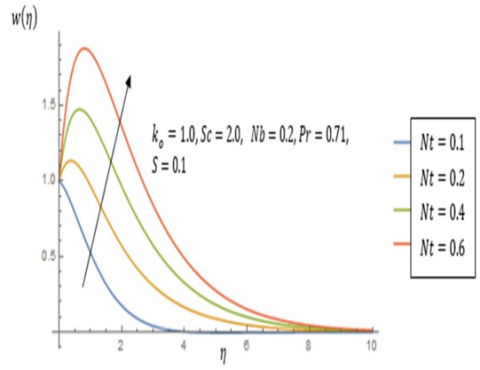Figure 17. Impact of thermophoresis number, Nt on nanoparticle (specie) concentration

## 18.png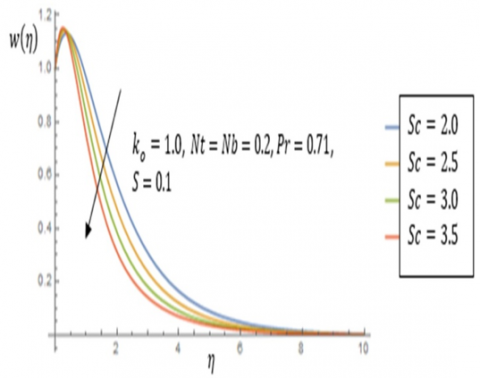Figure 18. Impact of Schmidt factor, $S c$ on nanoparticle (specie) concentration

## 19.png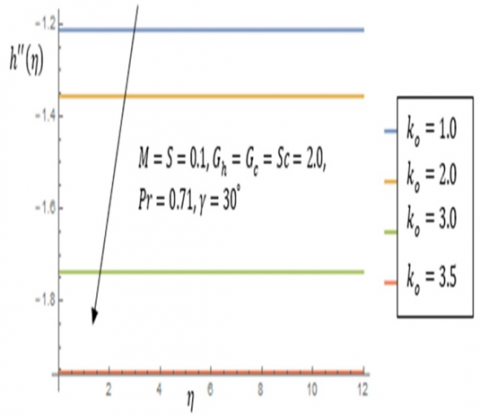Figure 19. Impact of velocity suction, $k_o$ on skin-friction

## 20.png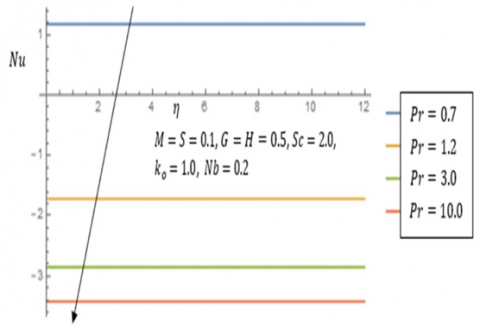Figure 20. Impact of Prandtl parameter, Pr on the rate of heat transfer

## 21.png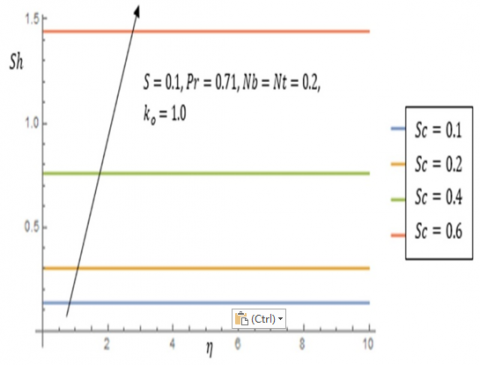Figure 21. Impact of Schmidt number, Sc on the rate of mass transfer

Table 1. Effect of suction velocity parameter, $k_o$ on skin-friction with $G_h=G_c=2.0$

 M S Pr Sc γ ko Cf 0.1 0.1 0.71 2.0 30° 1.0 -1.2126 0.1 0.1 0.71 2.0 30° 2.0 -1.3563 0.1 0.1 0.71 2.0 30° 3.0 -1.7375 0.1 0.1 0.71 2.0 30° 3.5 -1.9536

Table 2. Effect of Prandtl number, $P r$ on the rate of heat transmission as M=S=0.1

 Nb G H Pr Sc ko -Nu 0.2 0.5 0.5 0.7 2.0 1.0 1.1732 0.2 0.5 0.5 1.2 2.0 1.0 -1.7195 0.2 0.5 0.5 3.0 2.0 1.0 -2.8539 0.2 0.5 0.5 10.0 2.0 1.0 -3.4258

Table 3. Effect of Schmidt parameter, Sc on the rate of mass transmission at Nb=Nt=0.2

 S Pr Sc ko Sh 0.1 0.71 0.1 1.0 0.1339 0.1 0.71 0.2 1.0 0.3015 0.1 0.71 0.4 1.0 0.7581 0.1 0.71 0.6 1.0 1.4412
5. Discussion

Figures 2 to 7 denotes the rate of flow (velocity) distributions under various parameters of interest in the study of fluid. From the first figure, flow field declines as the magnetic number intensifies. This is evidenced due to drag which pulls the flowing speed of the nanofluid backward. Thus, this opposing force is termed Lorentz force. Therefore, in order to effectively control the fluid velocity in a given industrial mechanical systems, the magnetic force play a crucial role. In Figures 3 and 4, the effect of both modified convective heat and specie Grashof strengths $G_h, G_c$ are elaborated. As a result of the dynamics existing between buoyancy and viscid drag owing to free convection in the nanofluid, its rate of passage increases as these numbers improves. The influence of suction parameter, $k_o$ on the flow profile is shown in Figure 5. With the creation of artificial vacuum through the removal of air so as to force fluid into an empty space, suction is encountered. This is applicable in several medical processes in getting rid of respiratory secretions, removing of blood from the airways as well as other poisonous substances from the lungs of patients. Hence, the velocity of the fluid transmission retards. In Figure 6, the appreciation of radiation number, S resulting from both electric and magnetic energy leads to a rise in the flow field. On the other hand, the impact of the inclination angle, γ on the velocity of the nanoliquid is depicted in Figure 7. Expansion in the values of this factor begets a fall in fluid rapidity.

The representation of the effect of quantities on temperature are demonstrated from Figures 8-13. Thus, impartation of heat dependent non-uniform numbers G and H on the temperature is featured in Figure 8. Meanwhile, increase of heat supply in form of thermal energy sparks the degree of fluid hotness which in turn raises the temperature. Similarly, improvement in the values of velocity suction parameter, $k_o$ lowers the temperature and this is captured in Figure 9. The effect of radiation number, S on temperature is showcased in Figure 10. Due to the increment in electromagnetic wave vis-a-vis radiation from an external source, heat energy of the fluid will appreciate. In Figure 11, the rate of thermal absorption distribution heightens as the magnetic field quantity amplifies. Subsequently, the consequence of raising the nanoparticle (specie) random movement Nb on the temperature appears in Figure 12. The constant and zigzag motion experienced in the nanofluid by the nanoparticles, leads to an increase in its energetic and kinetic strength which is converted into mechanical energy thereby enhancing the degree of hotness of the nanofluid. Thus, this confirms the nanofluid as a good conductor of heat. Evolution of the result of intensifying values of the Prandtl parameter, Pr on the conducting nanofluid is featured in Figure 13. In the case of metals in liquid form, smaller values of Pr, signifies that thermal phenomenon disperses faster as a result of bigger wall layer thickness. Thus, this explains the greater heat conductivity property associated with such nanofluids. Therefore, the rate of fluids’ hotness declines with appreciation of Pr showing that it (Pr) plays an impressive role in taming the degree of fluid hotness. The impression of suction and radiation parameters ko and S on specie concentration are demonstrated in Figures 14 and 15 respectively. The direction of nanoparticle specie concentration decreases as both constraints are raised. Figures 16 and 17 uncovers the outcome on the specie flow field as the nanoparticle chaotic motion and thermomigration factors Nb and Nt expands. As the enhancement in the value of Nb breeds a decline in specie concentration, the reverse applies when there is an enhancement in Nt. Thus, the transporting of nanoparticles in a nanofluid rises in response to differences in temperature between the hot fluid and cold boundary. Figure 18, explains the rationale behind the Schmidt factor Sc, on nanoparticle concentration. As this number involves the relation of kinematic viscosity to mass diffusion, the specie concentration drops as coefficient of molecular diffusivity increases more than dynamic viscosity.

Appreciating values of $k_o$ indicates a fall in skin friction coefficient. This phenomenon is tabulated and pictured in Table 1 and Figure 19. The deterioration in the rate of thermal transfer as values of Pr intensifies is denoted by Table 2 and Figure 20. Similarly, as the rate of convection mass transmission outweighs the diffusivity molecular motion, the rate of mass transference number upsurges with a surge in Sc as shown in Table 3 and Figure 21 respectively.

6. Conclusion

The analytical and numerical simulation of nano-fluid transfusion over an inclined heated Sheet in the presence of temperature dependent non-uniform heat generation, radiation flux and hydro-magnetic is studied. The outcomes are reported in graphical and legend forms with the evolution of impacts of different physical fluid parameters. Based on our presented graphs, we realised the following findings from our analysis.

• The velocity profile shrinks as the suction, inclination angle and magnetic field numbers increases while it appreciates as radiation, convective heat and concentration Grashof numbers rise.
• The temperature improves with enhancement of thermal dependent non-uniform, magnetic field, radiation, and nanoparticle motion parameters enhances where as it depreciates by raising the Prandtl and suction factors.
• Improving effect of nanoparticle motion, thermodiffusion, radiation, Schmidt and suction parameters shows a decreasing concentration distribution but enlarges in behavior with enrichment of the thermodiffusion number.
• Skin friction depreciates due to augmentation of the suction parameter.
• The intensification of the Prandtl number leads to a decline of the Nusselt number.
• Sherwood number surges as the Schmidt number is augmented.
• For a more robust technological advancement, the application of conducting fluids (ferro-fluids) is paramount.
• The radiators of aumobiles serves as a medium for heat transferal due to the key role it plays in the cooling processes of the automobile engines for a better performance and life span extension.
• Medically, through the deployment of suction, the rate of fluid flow in the body of a patient with a certain health challenge can be controlled.
Nomenclature
 u, v component of non-dimensional velocities in x and y directions $\left(m s^{-2}\right)$ x, y coordinates of the horizontal and vertical axes U non-dimensional free stream velocity $\left(m s^{-1}\right)$ $U_w$ velocity at the surface of the plate $\left(m s^{-1}\right)$ $T_{\infty}$ free stream temperature $(K)$ $T_w$ temperature of stretched plate surface $(K)$ $C_w$ nanoparticle concentration at the plate $\left(\mathrm{kg} \mathrm{m}^{-3}\right)$ $C_{\infty}$ nanoparticle mass concentration far away from the plate $\left(\mathrm{kg} \mathrm{m}^{-3}\right)$ T temperature of the fluid $(K)$ $h^{\prime \prime}$ Skin friction $\mathrm{Nu}$ Local Nusselt number Sh Sherwood number $C_p$ heat capacity at constant pressure $\left(J k g^{-1} K^{-1}\right)$ $B_0^2$ even magnetic field strength $\left(\mathrm{Wb} m^{-3}\right)$ $D_T$ thermophoresis mass diffusion coefficient $\left(m^2 / s\right)$ $D_m$ molecular mass coefficient $\left(m^2 s^{-1}\right)$ $h^{\prime}$ Non-dimensional velocity $g$ Dimensionless temperature $w$ Non-dimensional concentration Greek symbols $\beta$ coefficient of convectional fluid volume enlargement t $\mathrm{t}\left(K^{-1}\right)$ $k$ heat conduction parameter $\left(W m^{-1} K^{-1}\right)$ $v$ kinematic viscosity $\left(m^2 m^{-1}\right)$ $\mu$ dynamic viscosity $\rho_f$ fluid density $\left(\mathrm{kgm}^{-3}\right)$ $(\rho c)_f$ heat capacity of fluid $(J / K)$ $(\rho c)_p$ nanoparticle heat capacity $(J / K)$ $\varphi$ stream function $\tau$ shearing stress $\left(N m^{-2}\right)$ $\eta$ similarity variable $\sigma$ electrical conductivity of nanofluid $\sigma_s$ Stefan Boltzmann proportionality constant $\left(\mathrm{Wm}^2 \mathrm{~K}^4\right) 5.6697 \times 10^{-8}$ Subscripts $n_p$ nanoparticle $f$ convectional fluid $n_f$ nanofluid Symbols for the physical quantities $G, H$ temperature dependent heat parameters $\mathrm{Pr}$ Prandtl number $S$ radiation parameter $S c$ Schmidt number $\mathrm{Nb}$ Nanoparticle specie random motion parameter $N t$ thermodiffusion parameter $G_h$ modified heat Grashof number $G_c$ modified concentration Grashof number $k_o$ suction parameter M Magnetic field parameter $\gamma$ inclination angle factor
References

 Godson, I., Raju, B., Lal, D.M., Wongwises, S. (2010). Enhancement of heat transfer using nanofluids-an overview. Renewable and Sustainable Energy Reviews, 14(2): 629-641. https://doi.org/10.1016/j.rser.2009.10.004

 Keblinski, P. (2009). Thermal conductivity of nanofluids in Thermal Nanosystems and Nanomaterials. Springer Berlin Heidelberg, 213-221

 Özerinç, S., Kakaç, S., Yazıcıoğlu, A.G. (2010). Enhanced thermal conductivity of nanofluids: A state-of-the-art review. Microfluidics and Nanofluidics, 8(2): 145-170. https://doi.org/10.1007/s10404-009-0524-4

 Wen, D., Lin, G., Vafaei, S., Zhang, K. (2009). Review of nanofluids for heat transfer applications. Particuology, 7(2): 141-150. https://doi.org/10.1016/j.partic.2009.01.007

 Liu, J.P., Fullerton, E., Gutfleisch, O., Sellmyer, D.J. (Eds.). (2009). Nanoscale magnetic materials and applications. Dordrecht Heidelberg London New York: Springer Science+ Business Media, LLC. https://doi.org/10.1007/978-0-387-85600-1

 Timko, M., Koneracka, M., Kopcansky, P., Ramchand, C.N., Vekas, L., Bica, D. (2004). Application of magnetisable complex systems in biomedicine. Czechoslovak Journal of Physics, 54(4): 599-606. https://doi.org/10.1007/s10582-004-0153-9

 Mader, H.S., Wolfbeis, O.S. (2010). Optical ammonia sensor based on up-converting luminescent nanoparticles. Analytical Chemistry, 82(12): 5002-5004. https://doi.org/10.1021/ac1007283

 Raj, K. (1996). Magnetic Fluids and Devices: A Commercial Survey. Magnetic fluids and Applications Handbook, Begell House, New York, 657-329.

 Liu, T., Cheng, Y., Yang, Z. (2005). Design optimization of seal structure for stealing liquid by magnetic fluids. Journal of Magnetism and Magnetic Materials, 289: 411-414. https://doi.org/10.1016/j.jmmm.2004.11.116

 Alfven, H. (1942). Existence of electromagnetic-hydrodynamic wave. Nature, 150: 405-406. https://doi.org/10.1038/150405d0

 Ferraro, V.C.A., Plumpton, C. (1967). An introduction to magnetic-fluids mechanics. Geophysical Journal International, 12: 543-544.

 Singh, N.P., Singh, A.K. (2000). MHD effects on heat and mass transfer in flow of viscous fluid with induced magnetic field. Int. J. Pure Appl Physics., 38: 182-189.

 Singh, N.P., Singh, A.K. (2007). MHD free convection and mass transfer flow past a flat plate. Arabian Journal for Science and Engineering, 32: 93-112.

 Lawal, K.K., Jibril, H.M. (2021). Impact of relative motion of a magnetic field on unsteady magnetohydrodynamic natural convection flow with a constant heat source/sink. Heat Transfer, 50(1): 487-507. https://doi.org/10.1002/htj.21888

 Ramakrishna, S.B., Thavada, S,K., Venkatachala, G.M., Bandaru, M. (2021). Impacts of chemical reaction, diffusion-thermo and radiation on unsteady natural convective flow past an inclined plate under aligned magnetic field. Biointerface Research in Applied Chemistry, 11: 13252-13267. https://doi.org/10.33263/BRIAC115.1325213267

 Ilias, M.R., Ismail, N.S., Ab Raji, N.H., Rawi, N.A., Shafie, S. (2020). Unsteady aligned MHD boundary layer flow and heat transfer of a magnetic nanofluids past an inclined plate. International Journal of Mechanical Engineering and Robotics Research, 9: 197-206. https://doi.org/ 10.18178/ijmerr.9.2.197-206

 Masood, S., Farooq, M., Ahmad, S. (2020). Description of viscous dissipation in magnetohydrodynamic flow of nanofluid: Applications of biomedical treatment. Advances in Mechanical Engineering, 12: 1-13. https://doi.org/10.1177/1687814020926359

 Ganga, B., Govindaraju, M., Hakeem, A.A. (2019). Effects of inclined magnetic field on entropy generation in nanofluid over a stretching sheet with partial slip and non-linear thermal radiation. Iranian Journal of Science and Technology, Transactions of Mechanical Engineering, 43(4): 707-718. https://doi.org/10.1007/s40997-018-0227-0

 Dar, A.A. (2020). Effect of thermal radiation, temperature jump and inclined magnetic field on the peristaltic transport of blood flow in an asymmetric channel with variable viscosity and heat absorption/generation. Iranian Journal of Science and Technology, Transactions of Mechanical Engineering, 45: 487-501. https://doi.org/10.1007//s40997-020-00349-6

 Selvarasu, M., Balamurugan, K. (2021). Effect of viscous dissipation ohmic heating and hall current on MHD free convection flow past an inclined porous plate in the presence of Dufour effect, heat source and chemical reaction. Science, Technology and Development, 10: 221-232.

 Parashar, P.J., Ahmed, N. (2021). Mass transfer effect on a rotating MHD transcient flow of liquids lead through a porous medium in presence of hall and ion slip current with radiation. Mathematical Modelling of Engineering Problems, 8: 134-141. https://doi.org/10.1115/1-4005749

 Jena, S., Dash, G.C., Mushra, S.R. (2018). Chemical reaction effect on MHD viscoelastic fluid flow over a vertical stretching sheet with heat source/sink. Ain Shams Engineering Journal, 9(4): 1205-1213. https://doi.org/10.1016/j.asej.2016.06.014

 Gurram, D., Balamurugan, K.S., Raju, V.C.C., Vedavathi, N. (2017). Effect of Chemical Reaction on MHD Casson Fluid flow past an Inclined Surface with Radiation. Skit Research Journal, 7: 53-59.

 Raju, R.S. (2017). Trsnsfer effects on an unsteady MHD mixed convective flow past a vertical plate with chemical reaction. Engineering Transaction, 65: 221-249.

 Venkateswarlu, M., Reddy, G.V.R., Lakshmi, D.V. (2015). Radiation effects on MHD boundary layer flow of liquid metal over a porous streching surface in porous medium with heat generation. Journal of the Korean Society for Industrial and Applied Mathematics, 19: 83-102. https://dx.doi.org/10.12941/jksiam.201519.083

 Bilal, M., Nazeer, M. (2020). Numerical analysis for the non-newtonian flow over stratified stretching/shrinking inclined sheet with the aligned magnetic field and non-linear convection. Archive of Applied Mechanics, 91: 949-964. https://doi.org/10.1007/s00419-020-01798-w

 Usharami, V., Selvaraj, A, Constance, A.R., Neel, A.A. (2021). Impact of magnetohydrodynamics stream past an exponentially inclined vertical plate of first order chemical response with variable mass diffusion and thermal radiation. Mathematical Modelling and Computational Science, 1292: 485-497. https://doi.org/10.1007/978-981-33-4389-4_45

 Hakeem, A.K.A., Bhose, G., Sait, M.Y.A., Nagaraj, V.G., Muhammad, M.R. (2017). Nonlinear studies on the effect on non-uniform heat generation/absorption on hydrodynamic flow of nanofluid over a vertical plate. Journal of Nonlinear Analysis, Modelling and Control, 22(1): 1-16. https://dx.doi.org/10.15388/NA2017.1.1

 Poddar, S., Islam, M.M., Ferdouse, J., Alam, M.M. (2021). Characteristical analysis of MHD heat and mass transfer dissipative and radiating flid flow with magnetic field induction and suction. SN Applied Sciences, 3: 470. https://doi.org/10.1007/s2452-021-04452-4

 Uka, U.A., Amos, E., Nwaigwe, C. (2022). Chemical reaction and thermal radiation effects on magnetohydrodynamic nanofluid flow past an exponentially stretching sheet. Theoretical Mathematics and Applications, 12(2): 1-19. https://doi.org/10.47260/tma/1221